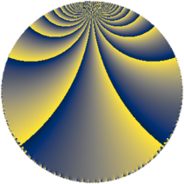# Properties

 Label 128.4.gLevel $128$ Weight $4$ Character orbit 128.g Rep. character $\chi_{128}(17,\cdot)$ Character field $\Q(\zeta_{8})$ Dimension $44$ Newform subspaces $1$ Sturm bound $64$ Trace bound $0$

# Related objects

## Defining parameters

 Level: $$N$$ $$=$$ $$128 = 2^{7}$$ Weight: $$k$$ $$=$$ $$4$$ Character orbit: $$[\chi]$$ $$=$$ 128.g (of order $$8$$ and degree $$4$$) Character conductor: $$\operatorname{cond}(\chi)$$ $$=$$ $$32$$ Character field: $$\Q(\zeta_{8})$$ Newform subspaces: $$1$$ Sturm bound: $$64$$ Trace bound: $$0$$

## Dimensions

The following table gives the dimensions of various subspaces of $$M_{4}(128, [\chi])$$.

Total New Old
Modular forms 208 52 156
Cusp forms 176 44 132
Eisenstein series 32 8 24

## Trace form

 $$44q + 4q^{3} - 4q^{5} + 4q^{7} - 4q^{9} + O(q^{10})$$ $$44q + 4q^{3} - 4q^{5} + 4q^{7} - 4q^{9} + 4q^{11} - 4q^{13} + 4q^{19} - 4q^{21} - 324q^{23} - 4q^{25} + 268q^{27} - 4q^{29} + 752q^{31} - 8q^{33} + 460q^{35} - 4q^{37} - 596q^{39} - 4q^{41} - 804q^{43} + 104q^{45} + 1384q^{51} + 748q^{53} + 292q^{55} - 4q^{57} - 1372q^{59} - 1828q^{61} - 2512q^{63} - 8q^{65} - 2036q^{67} - 1060q^{69} - 220q^{71} - 4q^{73} + 1712q^{75} + 1900q^{77} - 2436q^{83} + 496q^{85} + 1292q^{87} - 4q^{89} + 3604q^{91} - 112q^{93} + 6088q^{95} - 8q^{97} + 5424q^{99} + O(q^{100})$$

## Decomposition of $$S_{4}^{\mathrm{new}}(128, [\chi])$$ into newform subspaces

Label Dim. $$A$$ Field CM Traces $q$-expansion
$$a_2$$ $$a_3$$ $$a_5$$ $$a_7$$
128.4.g.a $$44$$ $$7.552$$ None $$0$$ $$4$$ $$-4$$ $$4$$

## Decomposition of $$S_{4}^{\mathrm{old}}(128, [\chi])$$ into lower level spaces

$$S_{4}^{\mathrm{old}}(128, [\chi]) \cong$$ $$S_{4}^{\mathrm{new}}(32, [\chi])$$$$^{\oplus 3}$$